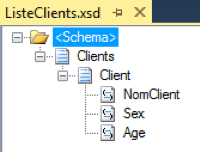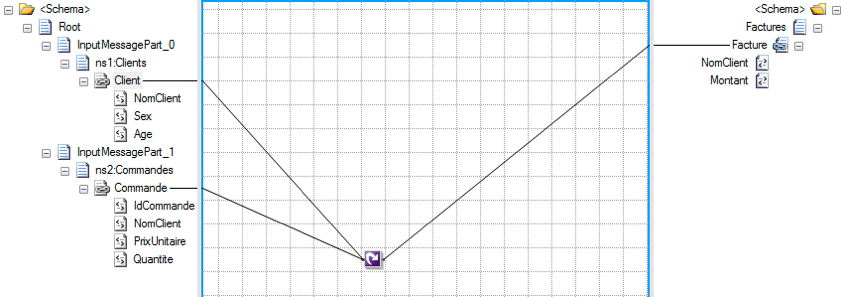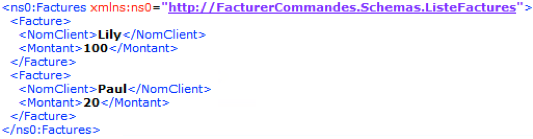# Embed loops in dual input mapCategory : BizTalk
12/11/2019

## CONTEXT

Recently, wanting to apply conditions to a dual input map where both source messages have repeating nodes, I realized that this was no easy matter.

### Situation

Message B (resulting from an SQL query) is a list of orders. Message A, meanwhile, is a new list of orders. The messages are definitely different, with two separate XSD models.

### Requirement

For each order in message A, the requirement is to browse the new list of orders and apply the following rule: if the order is not present in the new list, then it must be deleted (no further step is relevant here).

The problem arises when using the Looping functoid, whereby in coding an embedded loop on the two messages, the BizTalk mapper does not behave as desired. The mapper runs two loops separately on the outbound message and totally ignores our wish to run two embedded loops.

## DESCRIPTION OF A CASE STUDY

Taking a simplified example: the image below shows a list of clients, with the repeating Client node.The following shows a list of orders, with the repeating Order (Commande)node.The link between these 2 messages is the ClientName field (NomClient). Related rule is that for each order, if the ClientName field exists in the client list, the order is to be billed.

Outbound schema modeling the Invoice list is shown below:For the invoice amount, we simply multiply the UnitPrice (PrixUnitaire) and the Quantity (Quantite) fields.

We intuitively want to use the Looping functoid directly to read through our repeating nodes. We start by coding 2 loops on the 2 inbound schemas and analyzing the mapper’s behavior.The XSLT generated by the map is shown below:

```<xsl:template match="/s2:Root">
<ns0:Factures>
<xsl:for-each select="InputMessagePart_1/s0:Commandes/Commande">
<Facture />
</xsl:for-each>
<xsl:for-each select="InputMessagePart_0/s1:Clients/Client">
<Facture />
</xsl:for-each>
</ns0:Factures>
</xsl:template>```

The XSLT generated shows that we will have as many invoices as the sum total of orders plus clients. There is therefore no notion of embedded loops in this solution.

## Solution

There are two solutions to resolve this issue:

### Solution 1: using XSLT

As you might imagine, we can solve the problem using XSLT:

```<xsl:element name="ns0:Invoices" xmlns:ns0="http://InvoiceOrders.Schemas.InvoiceList">
<xsl:for-each select="/*[local-name()='Root']/*[local-name()='InputMessagePart_1']/*[local-name()='Orders']/*[local-name()='Order']">
<xsl:element name="Invoice">
<xsl:variable name="OrderClientName" select="string(./ClientName)" />
<xsl:variable name="findClient" select="count(/*[local-name()='Root']/*[local-name()='InputMessagePart_0']/*[local-name()='Clients']/*[local-name()='Client'][*[local-name()='ClientName' and text()=\$OrderClientName]])" />
<xsl:if test="\$findClient!=number(0)">
<xsl:element name="ClientName">
<xsl:value-of select="\$OrderClientName" />
</xsl:element>
<xsl:element name="Amount">
<xsl:variable name="Quantity" select="string(./Quantity)" />
<xsl:variable name="UPrice" select="string(./UnitPrice)" />
<xsl:value-of select="number(\$Quantity)*number(\$UPrice)" />
</xsl:element>
</xsl:if>
</xsl:element>
</xsl:for-each>
</xsl:element>
```

As you can see, the solution is to read through our list of orders and then search for the relevant client in the client list message. It’s that easy.

### Solution 2: with functoids

This solution is a little less intuitive to set up, but proves very useful in situations where you cannot produce your map in full XSLT (if the map already exists, or if the destination schema is highly complex, for example).

Here is what the map looks like:First of all, a loop has to be set up on the list of orders (see the Looping functoid). Next, the backbone of this solution lies in the use of the Cumulative Concatenate functoid. In fact, this functoid enables us to loop around the client list. Then, we will use a script to determine whether the value in the ClientName field in the Orders message is to be found in the client list. Here is the script:

```public static bool CheckClient(string ClientName, string ClientList)
{
string[] vals = ClientList.Split(',');
return vals.Contains(ClientName);
}
```

## Result

Assume our inbound message (dual input) is as follows:Thus, with these 2 solutions, the following result is obtained:I hope the 2 solutions presented in this article will be of help.